# 雷达原理（一）：雷达波形

### 目录

这个系列主要学习雷达的相关原理和其 m a t l a b matlab 实现。
参考书籍：《雷达系统设计 m a t l a b matlab 仿真》

## 1.低通，带通信号和正交分量

如果信号包含的主要频率处于包括直流 ( D C ) (DC) 在内的低频频带,则称其为低通 ( L P ) (LP) 信号。如果信号包含的主要频率处于离开原点的某个频率附近,则称其为带通 ( B P ) (BP) 信号。一个实带通信号 x ( t ) x( t) 在数学上可表示为:

x ( t ) = r ( t ) c o s ( 2 π f 0 t + ϕ x ( t ) ) x(t)=r(t)cos(2\pi f_{0}t+\phi_{x}(t))

f m ( t ) = 1 2 π d d t ϕ x ( t ) f_{m}(t)=\frac{1}{2\pi}\frac{d}{dt}\phi_{x}(t)

f i ( t ) = 1 2 π d d t ( 2 π f 0 t + ϕ x ( t ) ) = f 0 + f m f_{i}(t)=\frac{1}{2\pi}\frac{d}{dt}(2\pi f_{0}t+\phi_{x}(t))=f_{0}+f_{m}

x ( t ) = x I ( t ) c o s 2 π f 0 t − x Q ( t ) s i n 2 π f 0 t x(t)=x_{I}(t)cos2\pi f_{0}t-x_{Q}(t)sin2\pi f_{0}t

x I ( t ) = r ( t ) c o s ϕ x ( t ) x_{I}(t)=r(t)cos\phi_{x}(t)
x Q ( t ) = r ( t ) s i n ϕ x ( t ) x_{Q}(t)=r(t)sin\phi_{x}(t)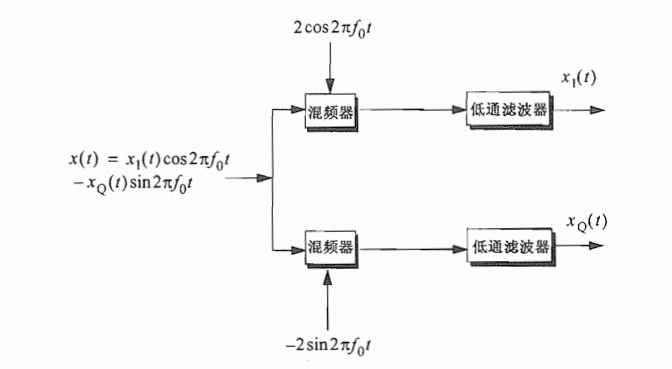## 2.解析信号

在上式中定义的正弦信号 x ( t ) x(t) 可以写为复信号的实部，更具体地可以表示为：

x ( t ) = R e x(t)=Re { ψ ( t ) ψ(t) }= R e Re { r ( t ) e j ϕ x ( t ) e j 2 π f o t r(t)e^{j\phi_{x}(t)e^{j2\pi f_{o}t}} }

ψ ( t ) = v ( t ) e j 2 π f 0 ( t ) ψ(t)=v(t)e^{j2\pi f_{0}(t)}

v ( t ) = r ( t ) j ϕ x ( t ) v(t)=r(t)^{j\phi_{x}(t)}

Ψ ( ω ) \quad\quad Ψ(ω) ψ ( t ) ψ(t) 的傅里叶变换， X ( ω ) X(ω) x ( t ) x(t) 的傅里叶变换。上式可以写为

Ψ ( ω ) = 2 U ( ω ) X ( ω ) Ψ(ω)=2U(\omega)X(\omega)

x ( t ) = u 0 I ( t ) c o s w 0 t − u 0 Q ( t ) s i n w 0 t x(t)=u_{0I}(t)cosw_{0}t-u_{0Q}(t)sinw_{0}t

x ( t ) = x I ( t ) c o s 2 π f 0 t − x Q ( t ) s i n 2 π f 0 t x(t)=x_{I}(t)cos2\pi f_{0}t-x_{Q}(t)sin2\pi f_{0}t

## 3.连续和脉冲波形

\quad 一个给定信号的频谱描述了其能量在频域的分布。一个能量信号(有限能量)的特征可以由它的能量谱密度( E S D ESD )函数来表示,而一个功率信号(有限功率)的特征可以由它的功率谱密度( P S D PSD )函数来表示。 E S D ESD 的单位为 J / H J/H , P S D PSD 的单位为 W / H W/H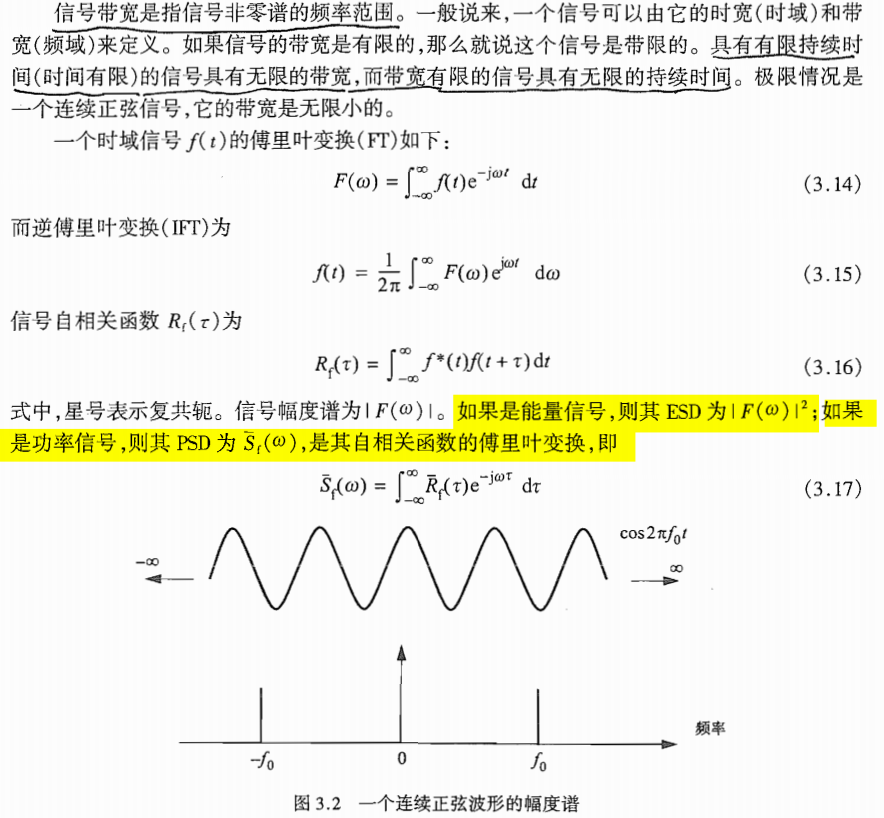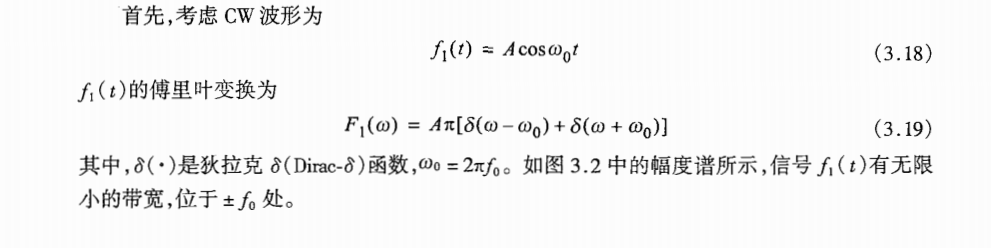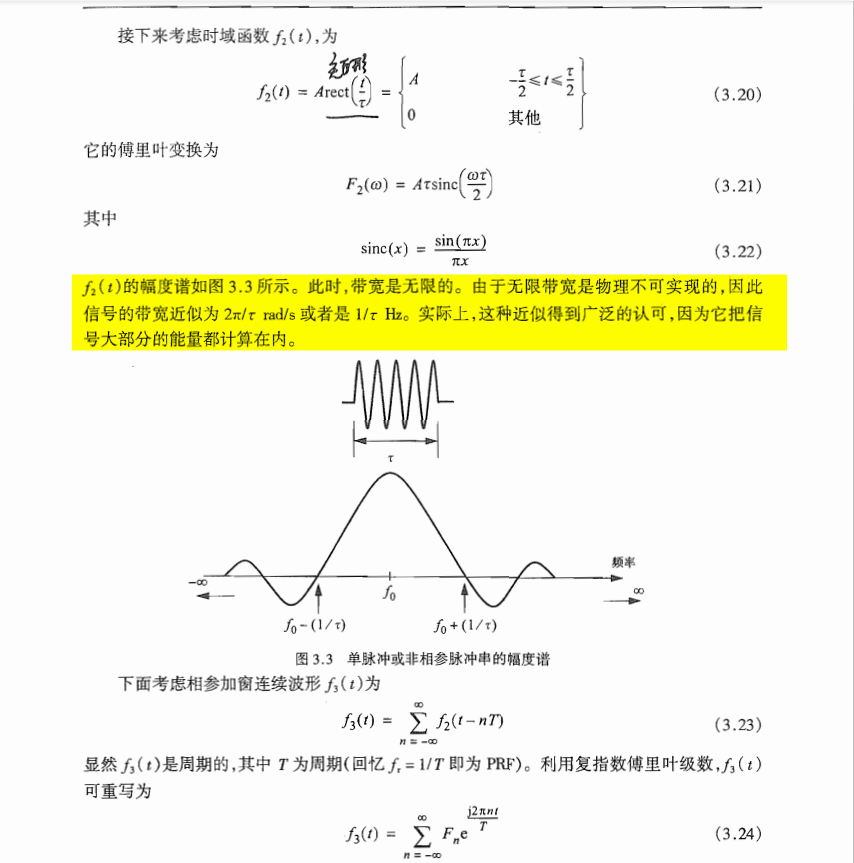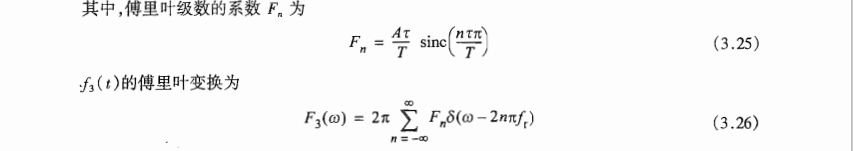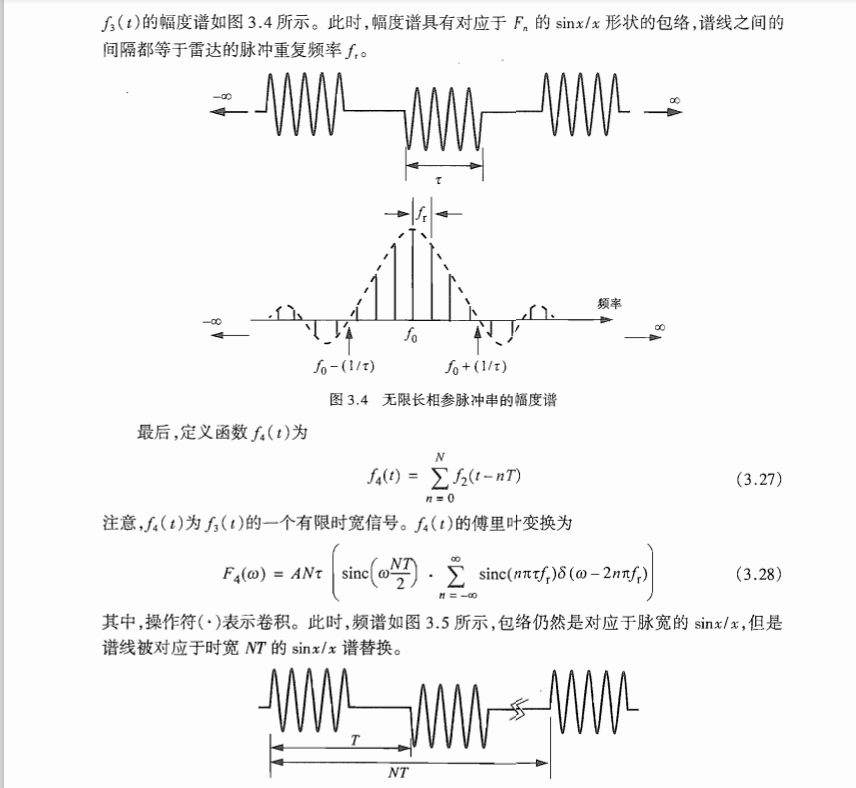## 4.线性调频波形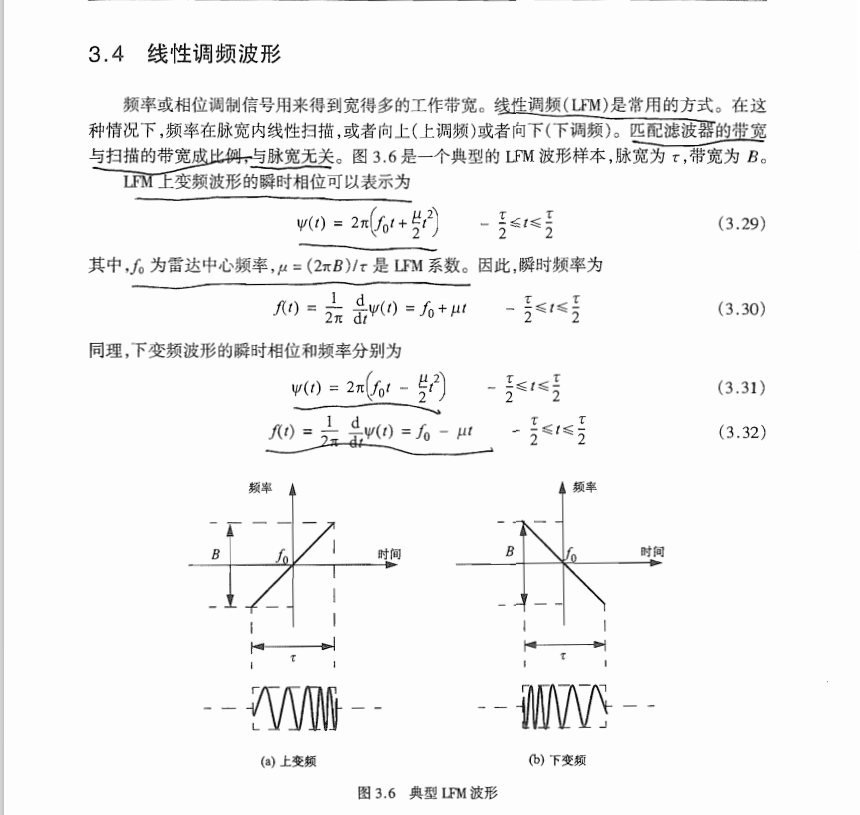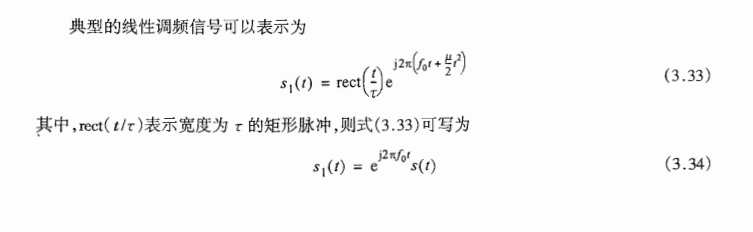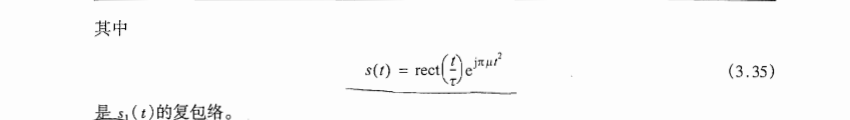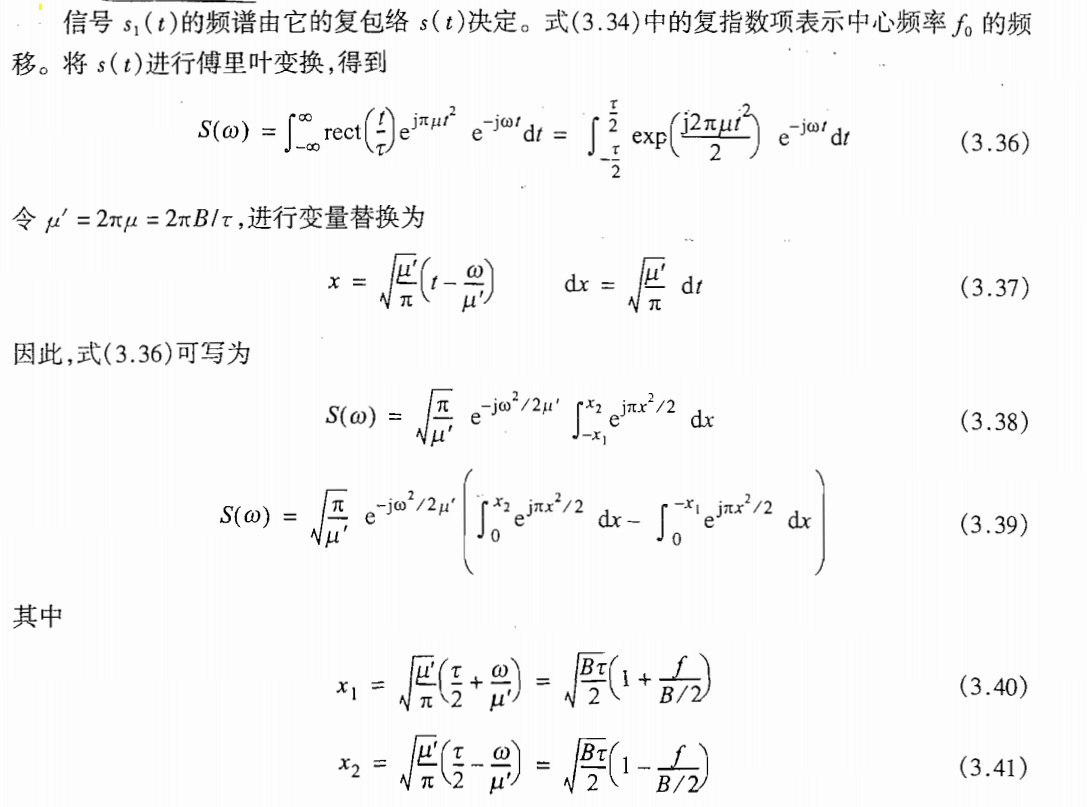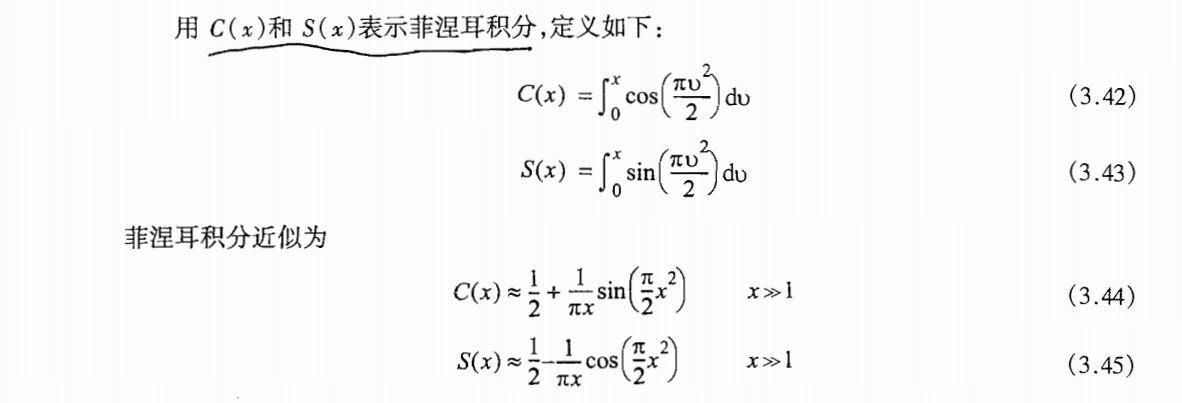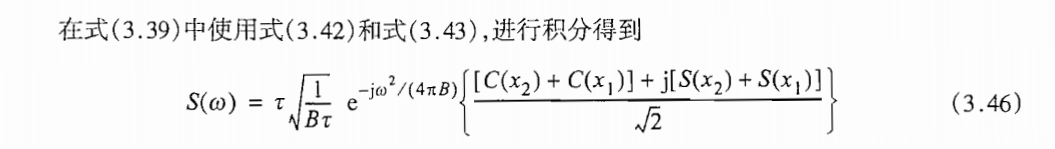function LFM(B,T);
time_B_product = B * T;
if(time_B_product < 5 )
fprintf('************ Time Bandwidth product is TOO SMALL ***************')
fprintf('\n Change B and or T')
return
else
end
% Compute alpha
mu = 2. * pi * B / T;
npoints = 5 * B * T + 1;
% Determine sampling times
delt = linspace(-T/2., T/2., npoints); %
% Compute the complex LFM representation
Ichannal = cos(mu .* delt.^2 / 2.); % Real part
Qchannal = sin(mu .* delt.^2 / 2.); % Imaginary Part
LFM = Ichannal + sqrt(-1) .* Qchannal; % complex signal
%Compute the FFT of the LFM waveform
LFMFFT = fftshift(fft(LFM));
% Plot the real and Inginary parts and the spectrum
sampling_interval = T / npoints;
freqlimit = 0.5 / sampling_interval;
freq = linspace(-freqlimit,freqlimit,npoints);
figure(1)
plot(delt,Ichannal,'k');
axis([-T/2 T/2 -1 1])
grid

xlabel('Time - seconds')
ylabel('Units of Waveform')
title('Real part of an LFM waveform')
figure(2)
plot(delt,Qchannal,'k');
axis([-T/2 T/2 -1 1])
grid

xlabel('Time - seconds')
ylabel('Units of Waveform')
title('Imaginary part of LFM waveform')
figure(3)
plot(freq, abs(LFMFFT),'k');
%axis tight
grid
xlabel('Frequency - Hz')
ylabel('Amplitude spectrum')
title('Spectrum for an LFM waveform')


## 5. 高距离分辨率

Δ R = c τ 2 = c 2 B ΔR=\frac{c\tau}{2}=\frac{c}{2B}

\quad\quad 雷达使用者和设计者不约而同地通过最小化 Δ R ΔR 来寻求实现高距离分辨率 ( H R R ) (HRR) 。然而如式(3.47)所示,为了得到高距离分辨率,必须使用短脉冲,从而导致平均发射功率的减小,并且强加了对大工作带宽的需求。在得到好的距离分辨率的同时保持足够的平均发射功率,可以通过使用脉冲压缩技术来实现,。利用频率或相位调制,脉冲压缩可以使我们获得相当长脉冲的平均发射功率,同时得到对应非常短脉冲的距离分辨率。例如,考虑一个 L F M LFM 波形,其带宽为B,未压缩脉宽为 τ \tau 。经过脉冲压缩后,压缩后的脉宽表示为 τ ′ \tau^{'} ，其中 τ ′ \tau^{'} << τ \tau , H R R HRR

Δ R = c τ ′ 2 < < c τ 2 ΔR=\frac{c\tau^{'}}{2}<<\frac{c\tau^{}}{2}

## 6.其他波形以后待补充（接着这篇文章添加）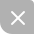UP更新不错过~
03-131万+
04-22
03-27222
04-152189
03-191506
04-26942
11-252090
12-161340
01-034214
03-03712
08-161万+
04-05
05-22
07-15
05-18
07-16788
01-093万+
09-132229

### “相关推荐”对你有帮助么？

•非常没帮助
•没帮助
•一般
•有帮助
•非常有帮助被折叠的  条评论 为什么被折叠?到【灌水乐园】发言¥2 ¥4 ¥6 ¥10 ¥20余额支付 (余额：-- )扫码支付获取中扫码支付点击重新获取扫码支付1.余额是钱包充值的虚拟货币，按照1:1的比例进行支付金额的抵扣。
2.余额无法直接购买下载，可以购买VIP、C币套餐、付费专栏及课程。余额充值